# From Wavelet Transforms to Convolutional Neural Networks - part 2¶

Author: Jean Feydy

In the second part of the workshop, we focus on a more realistic classification problem in which the FashionMNIST pieces of clothing are not perfectly centered. This is relevant as in practice, correct segmentation/extraction of image parts is at least as difficult as the classification of normalized+centered images...

In :
# PyTorch import
from __future__ import print_function
import torch
import torch.nn as nn
import torch.nn.functional as F
import torch.optim as optim
from torchvision import datasets, transforms, utils
import itertools

# Performance monitoring
from time import process_time
import matplotlib.pyplot as plt
import numpy as np
%matplotlib nbagg

# Disable warnings from the Scattering transform...
import warnings
warnings.filterwarnings("ignore")

# Train and visualize the performances of our models
from model_utils import AttrDict, show, generate_image, train, test, evaluate_model, display_classified_images
import model_utils as MU

MU.display_parameters()
# MU.use_cuda = False
# MU.args.epochs = 1 # If you just can't wait...

Optimizations will be made using the following arguments:
{'epochs': 10, 'log_interval': 10, 'test_batch_size': 250, 'batch_size': 250, 'momentum': 0.5, 'learning_rate': 0.01}
Using CUDA ?  Yes
Random seed :  1
Remember that you can change those values dynamically!
(MU.args.batch_size = ...)


Notice how we take advantage of the nice PyTorch syntax to apply randomized transformations every time an image is loaded.

In :
# Load the Fashion_MNIST dataset

DATASET = "./fashion_MNIST/" # Storage location
MU.class_names = ['T-shirt', 'Trouser', 'Pullover', 'Dress', 'Coat',
'Sandal',  'Shirt',   'Sneaker',  'Bag',   'Ankle boot' ]
datasets.MNIST.urls = [
'http://fashion-mnist.s3-website.eu-central-1.amazonaws.com/train-images-idx3-ubyte.gz',
'http://fashion-mnist.s3-website.eu-central-1.amazonaws.com/train-labels-idx1-ubyte.gz',
'http://fashion-mnist.s3-website.eu-central-1.amazonaws.com/t10k-images-idx3-ubyte.gz',
'http://fashion-mnist.s3-website.eu-central-1.amazonaws.com/t10k-labels-idx1-ubyte.gz'
]
kwargs = {'num_workers': 2, 'pin_memory': True} if MU.use_cuda else {}

MU.imgsize = (56,56)
MU.args.batch_size      = 250
MU.args.test_batch_size = 250

transform=transforms.Compose([
transforms.ToTensor(),
transforms.Normalize((0.1307,), (0.3081,)) # with normalized values
]))
MU.test_dataset  = datasets.MNIST(DATASET, train=False, transform=transforms.Compose([
transforms.ToTensor(),
transforms.Normalize((0.1307,), (0.3081,))
]))
# Iterator for the training pass:
batch_size=MU.args.batch_size, shuffle=True, # Data is drawn as randomized minibatches

# Iterator for the testing pass, with the same settings:
batch_size=MU.args.test_batch_size, shuffle=True,
**kwargs)

In :
# Display a few samples from the dataset.
# N.B.: since we've normalized the samples, this looks pretty ugly...

nrow = 4
images = [[] for i in range(10)]
for i in range(10000) :
images[MU.train_dataset[i]].append( MU.train_dataset[i] )
full = [len(l) >= nrow for l in images]
if all(full) :
break
show(utils.make_grid( list(itertools.chain(*[[ images[i][j] for j in range(nrow)] for i in range(10)])), nrow=nrow, normalize=True))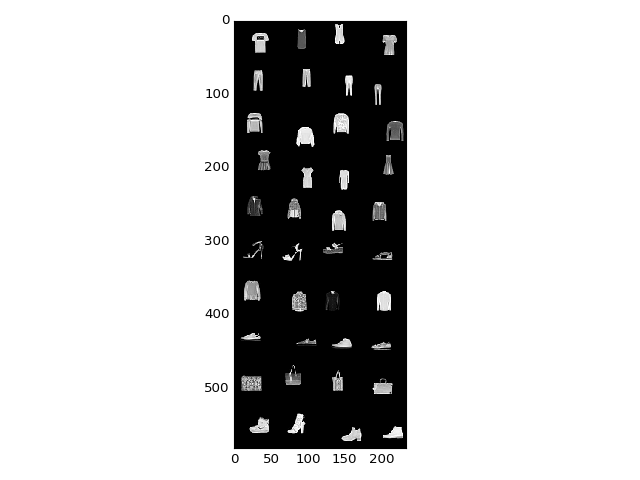# First try : a good old two-layer perceptron¶

In :
class TwoFullNet(nn.Module) :
"""
Implements a simplistic perceptron with 3 layers :
- one input, of size 28x28 (MNIST dataset)
- one hidden, of size N
- one output, of size 10 (number of classes)
There is no built-in regularization, and we model the two
transformations input->hidden and hidden->output as
Linear->ReLu and Linear->SoftMax operators,
i.e. as Fully connected computational graphs.
The trainable parameters are the weights of the matrices
(+ biases) involved in the "Linear" (Affine, really) operators.
"""
def __init__(self, N) :
"Defines the parameters of the model."
super(TwoFullNet, self).__init__()
# Linear (i.e. fully connected) layer, a matrix of size (28*28)xN
self.fc1        = nn.Linear(MU.imgsize*MU.imgsize, N)
# Linear (i.e. fully connected) layer, a matrix of size Nx10 (10 classes as output)
self.fc2        = nn.Linear( N, 10)

def forward(self, x) :
"""
Apply the model to some input data x.
You can think of x as an image of size 28x28, but it is
actually an Mx28x28 tensor, where M is the size of the
mini-batch.
"""
x = x.view(-1, MU.imgsize*MU.imgsize) # Turns our image into a vector
x = self.fc1( x )     # Linear transformation
x = F.relu(   x )     # Non-linearity (Relu = "positive part", a typical choice)
x = self.fc2( x )     # Second linear transformation
# Really, the softmax is the classification label, but for numerical stability,
# all computations are made in the log domain
return F.log_softmax(x)

two_fc_classifier = TwoFullNet(100)
if MU.use_cuda : two_fc_classifier.cuda()

In :
evaluate_model(two_fc_classifier)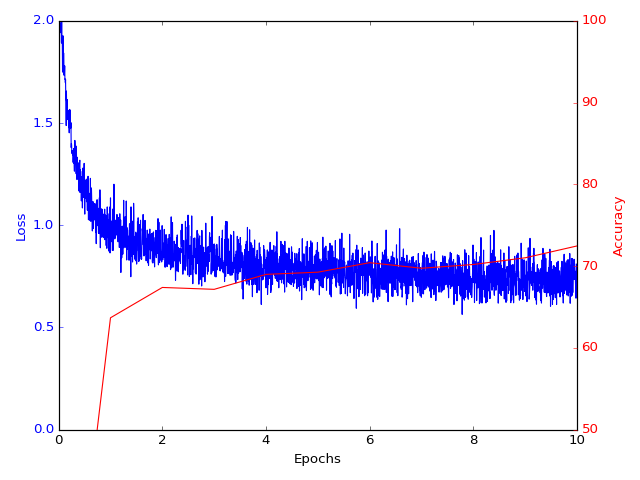Time Elapsed:    1.13s, Average test loss: 2.3130, Test accuracy: 1040/10000 (10%)
Time Elapsed:   60.22s, Average test loss: 0.9905, Test accuracy: 6368/10000 (64%)
Time Elapsed:  122.86s, Average test loss: 0.8792, Test accuracy: 6739/10000 (67%)
Time Elapsed:  183.52s, Average test loss: 0.8570, Test accuracy: 6716/10000 (67%)
Time Elapsed:  246.59s, Average test loss: 0.8228, Test accuracy: 6899/10000 (69%)
Time Elapsed:  307.37s, Average test loss: 0.8063, Test accuracy: 6926/10000 (69%)
Time Elapsed:  372.35s, Average test loss: 0.7800, Test accuracy: 7042/10000 (70%)
Time Elapsed:  437.89s, Average test loss: 0.7711, Test accuracy: 6974/10000 (70%)
Time Elapsed:  504.42s, Average test loss: 0.7779, Test accuracy: 7020/10000 (70%)
Time Elapsed:  576.92s, Average test loss: 0.7579, Test accuracy: 7102/10000 (71%)
Time Elapsed:  642.29s, Average test loss: 0.7361, Test accuracy: 7247/10000 (72%)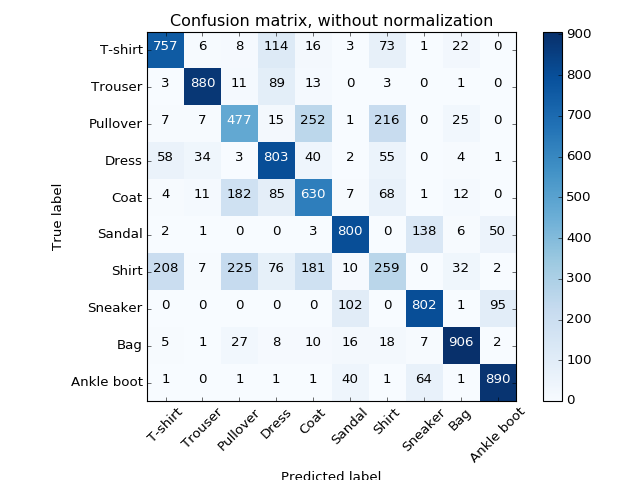Confusion matrix, without normalization


Performances are considerably lower than in the centered case. Was this expected?

In :
synt_img = generate_image(two_fc_classifier, mode = "class", target_class = 2, seed = 'zero')

fig = plt.figure() ; plt.imshow(synt_img, interpolation='nearest', cmap='gray') ;
plt.tight_layout() ; fig.canvas.draw()

Loss -0.00
Probas per class :  0%, 0%, 100%, 0%, 0%, 0%, 0%, 0%, 0%, 0%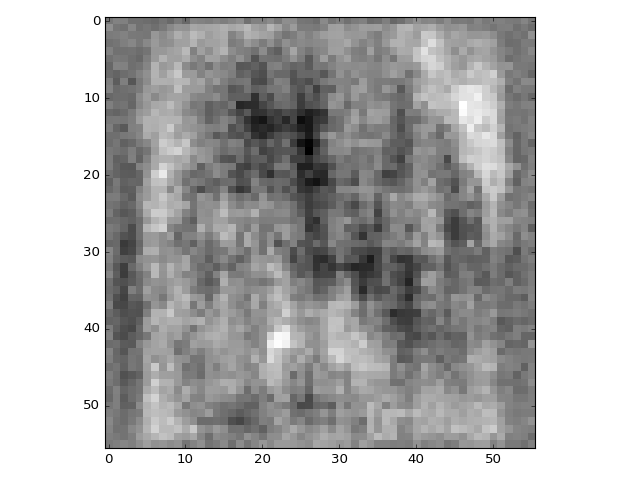In :
nrow = 10
images = [torch.Tensor(generate_image(two_fc_classifier, mode = "neuron",
target_operator = two_fc_classifier.fc1, target_neuron = (i,),
seed = 'zero', verbose=False)).expand(3,MU.imgsize,MU.imgsize)
for i in range(100)]
show(utils.make_grid( list(images), nrow=nrow, normalize=True))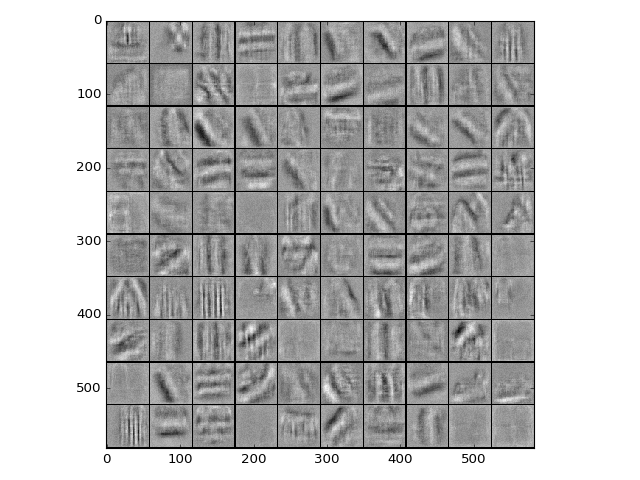Quite remarkably, the first operator of our model has converged towards a DiscreteCosinus-like Transform, made out of stripes! This makes sense, as a linear and translation-invariant operator is necessarily diagonal in the Fourier basis: even though it relies on non-linearities, our network has converged towards a kind of "spectral" translation-invariant classifier.

In :
# By the way : parameters of the second layer can be accessed too
print(two_fc_classifier.fc2.weight)
print(two_fc_classifier.fc2.bias)

Parameter containing:
-0.0122  0.1190 -0.1480  ...  -0.0365 -0.0021 -0.0311
0.1685 -0.1723  0.1454  ...  -0.0200  0.0284 -0.0725
-0.0421  0.0799 -0.2029  ...  -0.0703 -0.0107 -0.0897
...             ⋱             ...
0.0512 -0.0024  0.0640  ...   0.0071  0.0012 -0.0334
0.1307  0.1180 -0.0262  ...  -0.1511 -0.0230  0.0321
0.0602 -0.0110  0.1477  ...   0.1262 -0.0155 -0.0445
[torch.cuda.FloatTensor of size 10x100 (GPU 0)]

Parameter containing:
0.0829
-0.0661
0.0475
0.0567
-0.0780
0.0654
0.1490
-0.1175
0.0742
-0.0958
[torch.cuda.FloatTensor of size 10 (GPU 0)]



# Enforcing translation invariance : Convolutional Neural Networks¶

Expecting a generic perceptron to give perfect results was too optimistic: as it is theoretically able to emulate any classifier, it is prone to overfit the training data. In a sense, we already encountered such a problem in the Wavelet Thresholding Numerical Tour: http://nbviewer.jupyter.org/github/gpeyre/numerical-tours/blob/master/python/denoisingwav_2_wavelet_2d.ipynb

Replacing the orthogonal wavelet transform with a translation-invariant transform (using cycle-spinning or the algorithme à trous) dramatically increased the robustness of wavelet-based denoising algorithms; just the same, enforcing translation invariance in perceptrons will be a crucial step in the design of trainable operators for image processing. Fortunately, this prior is easy to enforce: we know that a translation-invariant linear operator can necessarily be represented as a convolution operator. It is thus natural to replace the generic linear operators known as "Fully connected layers" by their translation invariant counterparts, encoded as sets of convolution filters.

Furthermore, on top of translation invariance, we also know (or assume...) that natural images have a multiscale structure: there are relevant features at every scale, which are built as combinations of smaller details (edges -> eyes -> face). In practice, this means that we should prefer architectures with deep cascades of small convolution filters. Just like in a wavelet transform :-)

When designing a "neural network" (trainable transform) for image processing tasks, one thus typically restricts itself to a cascade of:

• Convolution operators such as nn.Conv2d.
• Su(b-p)sampling ("(un)pooling") operators such as F.max_pool2d.
• Pointwise operations such as F.relu.

(On top of this, several utility operators (batch normalization, dropout...) have also been developed but we won't detail them here.)

In :
class TwoConvTwoFullNet(nn.Module) :
"""
Implements a trainable model which is the concatenation
of two convolutional layers + two fully connected layers.
The choice of architecture here was mostly done at random,
for illustrative purpose...
"""
def __init__(self) :
super(TwoConvTwoFullNet, self).__init__()
# First conv operator : 30 1x5x5-filters + 30 bias constants
# which map an image of size WxH to a 3D volume of size 30xWxH
self.conv1      = nn.Conv2d( 1, 30, kernel_size=5)
# Second conv operator : 30 10x5x5-filters + 30 bias constants
# which map a 3D volume of size 30xWxH to a 3D volume of size 30xWxH
self.conv2      = nn.Conv2d(30, 30, kernel_size=5, groups=6)
# Dropout layer : probabilistic regularization trick
self.conv2_drop = nn.Dropout2d()
# Linear (i.e. fully connected) layer, a matrix of size (30*11*11)x100
self.fc1        = nn.Linear(30*11*11, 100)
# Linear (i.e. fully connected) layer, a matrix of size 100x10 (10 classes as output)
self.fc2        = nn.Linear( 100, 10)

def forward(self, x) :
"Stacks up the network layers, with the simplistic relu nonlinearity in-between."
x = F.max_pool2d(F.relu(                self.conv1(x)),  2)
x = F.max_pool2d(F.relu(self.conv2_drop(self.conv2(x))), 2)
# Up to this point, the treatment of x has been roughly translation-invariant:
# Conv2d operators and ReLu nonlinearities are completely T-I,
# whereas the subsampling "max_pool2d" operators are
# As we believe that the large-scale information should not be completely
# discarded (some features such as heels just happen to always be located in the bottom
# right corners of our images...), we end our pipeline (transform) with
# a regular two-layers perceptrons that processes the reduced image x
# as a features vector.

# At this point, x is a 3D volume of size 30xWxH.
# Due to convolution truncatures and subsamplings,
# we have W=H=11, so the following operation...
x = x.view(-1, 30*11*11)    # Turns it into a vector
x = F.relu(   self.fc1(x))  # 1x100 vector
x = F.dropout(x, training=self.training) # Add a dropout pass during training only
x = self.fc2( x)            # 1x10 vector
# Really, the softmax is the classification label, but for numerical stability,
# all computations are made in the log domain
return F.log_softmax(x)

two_layers_classifier = TwoConvTwoFullNet()
if MU.use_cuda : two_layers_classifier.cuda()

In :
evaluate_model(two_layers_classifier)